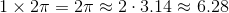Basic Geometry : Diameter

Example Questions

1 2 3 4 5 6 8 Next →

Example Question #376 : Basic Geometry

The diameter of a certain circle is tripled. Compared to the circumference of the original circle, how many times as large is the circumference of the new circle?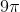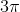Explanation:

The easiest way to find our answer is to try actual values. Imagine we have a circle with a diameter of. Given the formula for circumference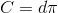our circumference is simply. Tripling the diameter gives a new diameter ofand therefore a new circumference of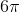. We can then determine the ratio between the two circumferences.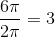Therefore, the new circumference is 3 times as large as the old.

Example Question #377 : Basic Geometry

Find the ratio of the diameter to the circumference in a circle with radius r.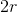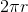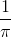Explanation:

To find a ratio, simply divide your two quantities. Remember,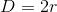Thus,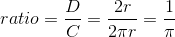Example Question #378 : Basic Geometry

What is the circumference to diameter ratio of a circle with a diameter of 15?

Cannot be determined.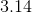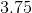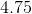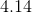Explanation:

First you must find the circumference of a circle with a diameter of 15 with the formula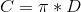.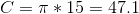Since the ratio of C to D is represented by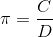, all of the ratios of Circumference to Diameter should approximately equal.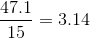Example Question #379 : Basic Geometry

In the game of Sumo, both wrestlers are placed on the outer edge of the ring, on opposite sides of each other. If the wrestling ring is a perfect circle and has an area of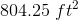, what would be the distance between both wrestlers?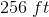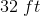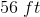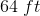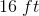Explanation:

With the two wrestlers standing opposite each other on the edge of the ring, the distance between them would constitute the diameter of the circle. We can use the formula for circular area to find the diameter: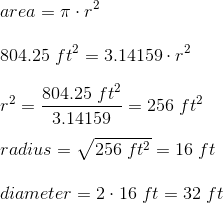Example Question #380 : Basic Geometry

True or false: A circle with radius 1 has circumference less than 4.

False

True

The circumference of a circle is equal to its radius multiplied by, so, if the radius of a circle is 1, its circumference is